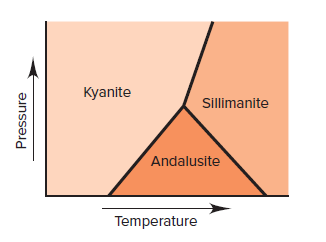# Problem: Kyanite, sillimanite, and andalusite all have the formula Al 2SiO5. Each is stable under different conditions (see the graph below). At the point where the three phases intersect:(b) Which mineral, if any, has the lowest enthalpy?

###### FREE Expert Solution
79% (387 ratings)
###### Problem Details

Kyanite, sillimanite, and andalusite all have the formula Al 2SiO5. Each is stable under different conditions (see the graph below). At the point where the three phases intersect:(b) Which mineral, if any, has the lowest enthalpy?

Frequently Asked Questions

What scientific concept do you need to know in order to solve this problem?

Our tutors have indicated that to solve this problem you will need to apply the Gibbs Free Energy concept. You can view video lessons to learn Gibbs Free Energy. Or if you need more Gibbs Free Energy practice, you can also practice Gibbs Free Energy practice problems.

What textbook is this problem found in?

Our data indicates that this problem or a close variation was asked in Chemistry: The Molecular Nature of Matter and Change - Silberberg 8th Edition. You can also practice Chemistry: The Molecular Nature of Matter and Change - Silberberg 8th Edition practice problems.